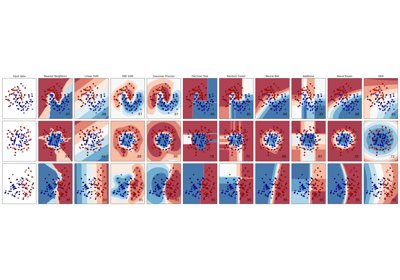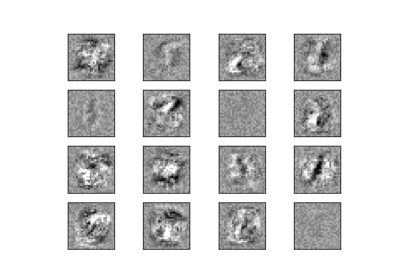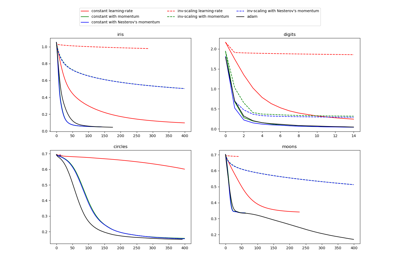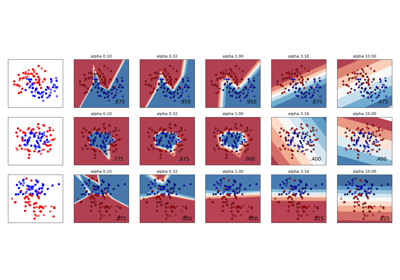# sklearn.neural_network.MLPClassifier¶

class sklearn.neural_network.MLPClassifier(hidden_layer_sizes=100, activation='relu', *, solver='adam', alpha=0.0001, batch_size='auto', learning_rate='constant', learning_rate_init=0.001, power_t=0.5, max_iter=200, shuffle=True, random_state=None, tol=0.0001, verbose=False, warm_start=False, momentum=0.9, nesterovs_momentum=True, early_stopping=False, validation_fraction=0.1, beta_1=0.9, beta_2=0.999, epsilon=1e-08, n_iter_no_change=10, max_fun=15000)[source]

Multi-layer Perceptron classifier.

This model optimizes the log-loss function using LBFGS or stochastic gradient descent.

New in version 0.18.

Parameters
hidden_layer_sizestuple, length = n_layers - 2, default=(100,)

The ith element represents the number of neurons in the ith hidden layer.

activation{‘identity’, ‘logistic’, ‘tanh’, ‘relu’}, default=’relu’

Activation function for the hidden layer.

• ‘identity’, no-op activation, useful to implement linear bottleneck, returns f(x) = x

• ‘logistic’, the logistic sigmoid function, returns f(x) = 1 / (1 + exp(-x)).

• ‘tanh’, the hyperbolic tan function, returns f(x) = tanh(x).

• ‘relu’, the rectified linear unit function, returns f(x) = max(0, x)

The solver for weight optimization.

• ‘lbfgs’ is an optimizer in the family of quasi-Newton methods.

• ‘sgd’ refers to stochastic gradient descent.

• ‘adam’ refers to a stochastic gradient-based optimizer proposed by Kingma, Diederik, and Jimmy Ba

Note: The default solver ‘adam’ works pretty well on relatively large datasets (with thousands of training samples or more) in terms of both training time and validation score. For small datasets, however, ‘lbfgs’ can converge faster and perform better.

alphafloat, default=0.0001

L2 penalty (regularization term) parameter.

batch_sizeint, default=’auto’

Size of minibatches for stochastic optimizers. If the solver is ‘lbfgs’, the classifier will not use minibatch. When set to “auto”, batch_size=min(200, n_samples)

Learning rate schedule for weight updates.

• ‘constant’ is a constant learning rate given by ‘learning_rate_init’.

• ‘invscaling’ gradually decreases the learning rate at each time step ‘t’ using an inverse scaling exponent of ‘power_t’. effective_learning_rate = learning_rate_init / pow(t, power_t)

• ‘adaptive’ keeps the learning rate constant to ‘learning_rate_init’ as long as training loss keeps decreasing. Each time two consecutive epochs fail to decrease training loss by at least tol, or fail to increase validation score by at least tol if ‘early_stopping’ is on, the current learning rate is divided by 5.

Only used when solver='sgd'.

learning_rate_initdouble, default=0.001

The initial learning rate used. It controls the step-size in updating the weights. Only used when solver=’sgd’ or ‘adam’.

power_tdouble, default=0.5

The exponent for inverse scaling learning rate. It is used in updating effective learning rate when the learning_rate is set to ‘invscaling’. Only used when solver=’sgd’.

max_iterint, default=200

Maximum number of iterations. The solver iterates until convergence (determined by ‘tol’) or this number of iterations. For stochastic solvers (‘sgd’, ‘adam’), note that this determines the number of epochs (how many times each data point will be used), not the number of gradient steps.

shufflebool, default=True

Whether to shuffle samples in each iteration. Only used when solver=’sgd’ or ‘adam’.

random_stateint, RandomState instance, default=None

Determines random number generation for weights and bias initialization, train-test split if early stopping is used, and batch sampling when solver=’sgd’ or ‘adam’. Pass an int for reproducible results across multiple function calls. See Glossary.

tolfloat, default=1e-4

Tolerance for the optimization. When the loss or score is not improving by at least tol for n_iter_no_change consecutive iterations, unless learning_rate is set to ‘adaptive’, convergence is considered to be reached and training stops.

verbosebool, default=False

Whether to print progress messages to stdout.

warm_startbool, default=False

When set to True, reuse the solution of the previous call to fit as initialization, otherwise, just erase the previous solution. See the Glossary.

momentumfloat, default=0.9

Momentum for gradient descent update. Should be between 0 and 1. Only used when solver=’sgd’.

nesterovs_momentumbool, default=True

Whether to use Nesterov’s momentum. Only used when solver=’sgd’ and momentum > 0.

early_stoppingbool, default=False

Whether to use early stopping to terminate training when validation score is not improving. If set to true, it will automatically set aside 10% of training data as validation and terminate training when validation score is not improving by at least tol for n_iter_no_change consecutive epochs. The split is stratified, except in a multilabel setting. Only effective when solver=’sgd’ or ‘adam’

validation_fractionfloat, default=0.1

The proportion of training data to set aside as validation set for early stopping. Must be between 0 and 1. Only used if early_stopping is True

beta_1float, default=0.9

Exponential decay rate for estimates of first moment vector in adam, should be in [0, 1). Only used when solver=’adam’

beta_2float, default=0.999

Exponential decay rate for estimates of second moment vector in adam, should be in [0, 1). Only used when solver=’adam’

epsilonfloat, default=1e-8

n_iter_no_changeint, default=10

Maximum number of epochs to not meet tol improvement. Only effective when solver=’sgd’ or ‘adam’

New in version 0.20.

max_funint, default=15000

Only used when solver=’lbfgs’. Maximum number of loss function calls. The solver iterates until convergence (determined by ‘tol’), number of iterations reaches max_iter, or this number of loss function calls. Note that number of loss function calls will be greater than or equal to the number of iterations for the MLPClassifier.

New in version 0.22.

Attributes
classes_ndarray or list of ndarray of shape (n_classes,)

Class labels for each output.

loss_float

The current loss computed with the loss function.

best_loss_float

The minimum loss reached by the solver throughout fitting.

loss_curve_list of shape (n_iter_,)

The ith element in the list represents the loss at the ith iteration.

t_int

The number of training samples seen by the solver during fitting.

coefs_list of shape (n_layers - 1,)

The ith element in the list represents the weight matrix corresponding to layer i.

intercepts_list of shape (n_layers - 1,)

The ith element in the list represents the bias vector corresponding to layer i + 1.

n_iter_int

The number of iterations the solver has ran.

n_layers_int

Number of layers.

n_outputs_int

Number of outputs.

out_activation_str

Name of the output activation function.

loss_curve_list of shape (n_iters,)

Loss value evaluated at the end of each training step.

t_int

Mathematically equals n_iters * X.shape, it means time_step and it is used by optimizer’s learning rate scheduler.

Notes

MLPClassifier trains iteratively since at each time step the partial derivatives of the loss function with respect to the model parameters are computed to update the parameters.

It can also have a regularization term added to the loss function that shrinks model parameters to prevent overfitting.

This implementation works with data represented as dense numpy arrays or sparse scipy arrays of floating point values.

References

Hinton, Geoffrey E.

“Connectionist learning procedures.” Artificial intelligence 40.1 (1989): 185-234.

Glorot, Xavier, and Yoshua Bengio. “Understanding the difficulty of

training deep feedforward neural networks.” International Conference on Artificial Intelligence and Statistics. 2010.

He, Kaiming, et al. “Delving deep into rectifiers: Surpassing human-level

performance on imagenet classification.” arXiv preprint arXiv:1502.01852 (2015).

Kingma, Diederik, and Jimmy Ba. “Adam: A method for stochastic

optimization.” arXiv preprint arXiv:1412.6980 (2014).

Examples

>>> from sklearn.neural_network import MLPClassifier
>>> from sklearn.datasets import make_classification
>>> from sklearn.model_selection import train_test_split
>>> X, y = make_classification(n_samples=100, random_state=1)
>>> X_train, X_test, y_train, y_test = train_test_split(X, y, stratify=y,
...                                                     random_state=1)
>>> clf = MLPClassifier(random_state=1, max_iter=300).fit(X_train, y_train)
>>> clf.predict_proba(X_test[:1])
array([[0.038..., 0.961...]])
>>> clf.predict(X_test[:5, :])
array([1, 0, 1, 0, 1])
>>> clf.score(X_test, y_test)
0.8...


Methods

 fit(X, y) Fit the model to data matrix X and target(s) y. get_params([deep]) Get parameters for this estimator. Predict using the multi-layer perceptron classifier Return the log of probability estimates. Probability estimates. score(X, y[, sample_weight]) Return the mean accuracy on the given test data and labels. set_params(**params) Set the parameters of this estimator.
fit(X, y)[source]

Fit the model to data matrix X and target(s) y.

Parameters
Xndarray or sparse matrix of shape (n_samples, n_features)

The input data.

yndarray, shape (n_samples,) or (n_samples, n_outputs)

The target values (class labels in classification, real numbers in regression).

Returns
selfreturns a trained MLP model.
get_params(deep=True)[source]

Get parameters for this estimator.

Parameters
deepbool, default=True

If True, will return the parameters for this estimator and contained subobjects that are estimators.

Returns
paramsmapping of string to any

Parameter names mapped to their values.

property partial_fit

Update the model with a single iteration over the given data.

Parameters
X{array-like, sparse matrix}, shape (n_samples, n_features)

The input data.

yarray-like, shape (n_samples,)

The target values.

classesarray, shape (n_classes), default None

Classes across all calls to partial_fit. Can be obtained via np.unique(y_all), where y_all is the target vector of the entire dataset. This argument is required for the first call to partial_fit and can be omitted in the subsequent calls. Note that y doesn’t need to contain all labels in classes.

Returns
selfreturns a trained MLP model.
predict(X)[source]

Predict using the multi-layer perceptron classifier

Parameters
X{array-like, sparse matrix} of shape (n_samples, n_features)

The input data.

Returns
yndarray, shape (n_samples,) or (n_samples, n_classes)

The predicted classes.

predict_log_proba(X)[source]

Return the log of probability estimates.

Parameters
Xndarray of shape (n_samples, n_features)

The input data.

Returns
log_y_probndarray of shape (n_samples, n_classes)

The predicted log-probability of the sample for each class in the model, where classes are ordered as they are in self.classes_. Equivalent to log(predict_proba(X))

predict_proba(X)[source]

Probability estimates.

Parameters
X{array-like, sparse matrix} of shape (n_samples, n_features)

The input data.

Returns
y_probndarray of shape (n_samples, n_classes)

The predicted probability of the sample for each class in the model, where classes are ordered as they are in self.classes_.

score(X, y, sample_weight=None)[source]

Return the mean accuracy on the given test data and labels.

In multi-label classification, this is the subset accuracy which is a harsh metric since you require for each sample that each label set be correctly predicted.

Parameters
Xarray-like of shape (n_samples, n_features)

Test samples.

yarray-like of shape (n_samples,) or (n_samples, n_outputs)

True labels for X.

sample_weightarray-like of shape (n_samples,), default=None

Sample weights.

Returns
scorefloat

Mean accuracy of self.predict(X) wrt. y.

set_params(**params)[source]

Set the parameters of this estimator.

The method works on simple estimators as well as on nested objects (such as pipelines). The latter have parameters of the form <component>__<parameter> so that it’s possible to update each component of a nested object.

Parameters
**paramsdict

Estimator parameters.

Returns
selfobject

Estimator instance.

## Examples using sklearn.neural_network.MLPClassifier¶